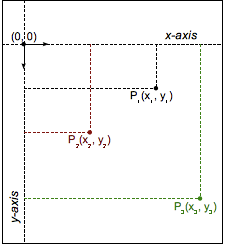/CSS

# transform-function()

The `<transform-function>` CSS data type represents a transformation that affects an element's appearance. Transformation functions can rotate, resize, distort, or move an element in 2D or 3D space. It is used in the `transform` property.

## Describing transformations mathematically

Various coordinate models can be used to describe an HTML element's size and shape, as well as any transformations applied to it. The most common is the Cartesian coordinate system, although homogeneous coordinates are also sometimes used.

###Cartesian coordinates

In the Cartesian coordinate system, a two-dimensional point is described using two values: an x coordinate (abscissa) and a y coordinate (ordinate). This is represented by the vector notation `(x, y)`.

In CSS (and most computer graphics), the origin `(0, 0)` represents the top-left corner of any element. Positive coordinates are down and to the right of the origin, while negative ones are up and to the left. Thus, a point that's 2 units to the right and 5 units down would be `(2, 5)`, while a point 3 units to the left and 12 units up would be `(-3, -12)`.

### Transformation functions

Transformation functions alter the appearance of an element by manipulating the values of its coordinates. A linear transformation function is described using a 2x2 matrix, like this:

$\left(\begin{array}{cc}a& c\\ b& d\end{array}\right)$

The function is applied to an element by using matrix multiplication. Thus, each coordinate changes based on the values in the matrix:

$\left(\begin{array}{cc}a& c\\ b& d\end{array}\right)\left(\begin{array}{cc}x& y\end{array}\right)=\left(\begin{array}{c}ax+cy\\ bx+dy\end{array}\right)$

It is even possible to apply several transformations in a row:

$\left(\begin{array}{cc}{a}_{1}& {c}_{1}\\ {b}_{1}& {d}_{1}\end{array}\right)\left(\begin{array}{cc}{a}_{2}& {c}_{2}\\ {b}_{2}& {d}_{2}\end{array}\right)=\left(\begin{array}{cc}{a}_{1}{a}_{2}+{c}_{1}{b}_{2}& {a}_{1}{c}_{2}+{c}_{1}{d}_{2}\\ {b}_{1}{a}_{2}+{d}_{1}{b}_{2}& {b}_{1}{c}_{2}+{d}_{1}{d}_{2}\end{array}\right)$

With this notation, it is possible to describe, and therefore compose, most common transformations: rotations, scaling, or skewing. (In fact, all transformations that are linear functions can be described.) Composite transformations are effectively applied in order from right to left.

However, one major transformation is not linear, and therefore must be special-cased when using this notation: translation. The translation vector `(tx, ty)` must be expressed separately, as two additional parameters.

Note: Though trickier than Cartesian coordinates, homogeneous coordinates in projective geometry lead to 3x3 transformation matrices, and can simply express translations as linear functions.

## Syntax

The `<transform-function>` data type is specified using one of the transformation functions listed below. Each function applies a geometric operation in either 2D or 3D.

### Matrix transformation

`matrix()`
Describes a homogeneous 2D transformation matrix.
`matrix3d()`
Describes a 3D transformation as a 4x4 homogeneous matrix.

### Perspective

`perspective()`
Sets the distance between the user and the z=0 plane.

### Rotation

`rotate()`
Rotates an element around a fixed point on the 2D plane.
`rotate3d()`
Rotates an element around a fixed axis in 3D space.
`rotateX()`
Rotates an element around the horizontal axis.
`rotateY()`
Rotates an element around the vertical axis.
`rotateZ()`
Rotates an element around the z-axis.

### Scaling (resizing)

`scale()`
Scales an element up or down on the 2D plane.
`scale3d()`
Scales an element up or down in 3D space.
`scaleX()`
Scales an element up or down horizontally.
`scaleY()`
Scales an element up or down vertically.
`scaleZ()`
Scales an element up or down along the z-axis.

### Skewing (distortion)

`skew()`
Skews an element on the 2D plane.
`skewX()`
Skews an element in the horizontal direction.
`skewY()`
Skews an element in the vertical direction.

### Translation (moving)

`translate()`
Translates an element on the 2D plane.
`translate3d()`
Translates an element in 3D space.
`translateX()`
Translates an element horizontally.
`translateY()`
Translates an element vertically.
`translateZ()`
Translates an element along the z-axis.

## Specifications

Specification Status Comment
CSS Transforms Level 1
The definition of 'transform' in that specification.
Working Draft Initial definition.

## Browser compatibilityUpdate compatibility data on GitHub

Desktop
Chrome Edge Firefox Internet Explorer Opera Safari
Basic support Yes ? 3.5
3.5
Firefox 14 removed experimental support for `skew()`, but it was reintroduced in Firefox 15.
Before Firefox 16, the translation values of `matrix()` and `matrix3d()` could be `<length>`s, in addition to the standard `<number>`.
9
9
Internet Explorer 9 supports 2D but not 3D transforms. In version 9, mixing 2D and 3D transform functions invalidates the entire property.
10.5 3.1
3D support 12 ? 10 10 15 4
Mobile
Android webview Chrome for Android Edge Mobile Firefox for Android Opera for Android iOS Safari Samsung Internet
Basic support 2 Yes ? Yes 11.5 3.2 Yes
3D support 3 Yes ? Yes 22 3.2 Yes